Courses

# MCQ : Pair Of Linear Equations In Two Variables - 1

## 10 Questions MCQ Test Mathematics (Maths) Class 10 | MCQ : Pair Of Linear Equations In Two Variables - 1

Description
This mock test of MCQ : Pair Of Linear Equations In Two Variables - 1 for Class 10 helps you for every Class 10 entrance exam. This contains 10 Multiple Choice Questions for Class 10 MCQ : Pair Of Linear Equations In Two Variables - 1 (mcq) to study with solutions a complete question bank. The solved questions answers in this MCQ : Pair Of Linear Equations In Two Variables - 1 quiz give you a good mix of easy questions and tough questions. Class 10 students definitely take this MCQ : Pair Of Linear Equations In Two Variables - 1 exercise for a better result in the exam. You can find other MCQ : Pair Of Linear Equations In Two Variables - 1 extra questions, long questions & short questions for Class 10 on EduRev as well by searching above.
QUESTION: 1

### If a pair of linear equations has infinitely many solutions, then the lines representing them will be

Solution:

If two lines are coincident then, they have infinite solution and a pair of linear equations is consistent; If two lines are intersecting then, they have a unique solution and a pair of linear equations is consistent. If we draw a rough graph of both the lines (as shown below) we will find that both lines are parallel and they have no solution, pair of linear equations is inconsistent.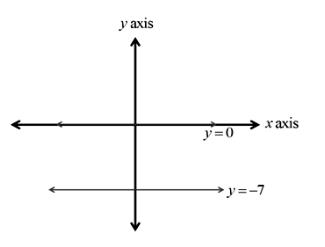QUESTION: 2

### The pair of linear equations 2x + 3y = 5 and 4x + 6y = 10 is

Solution:

a1 / a2 = b1 / b2 = c1 / c2
2/4 = 3/6 = 5/10
1/2 = 1/2 = 1/2
So, a1 / a2 = b1 / b2 = c1 / c2
When these are equal then it is consistent.
Therefore option (C) is correct .

QUESTION: 3

### If am ≠ bl, then the system of equations, ax + by = c, lx + my = n

Solution:

If am ≠ bl, then the equations ax+by=c, lx+my=n has a unique solution.

Given,

Pair of lines represented by the equations

ax + by = c

lx + my = n

For unique solution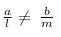For infinite solutions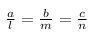For no solution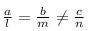Given,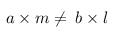This can be transformed into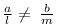Therefore, If am ≠ bl, then the equations ax+by=c, lx+my=n has a unique solution.

QUESTION: 4

The pair of equations y = 0 and y = - 7 has

Solution:

The equation are y=0 and y=-7
y=0 is on the x-axis and y=-7 is the line parallel to the x-axes at a distance 7 units from y=0
The line will be parallel
if we try to solve these equations we get 0=7 which is absurd.
So the equations are inconsistent.
Therefore there is no solution.

QUESTION: 5

The father’s age is six times his son’s age. Four years hence, the age of the father will be four times his son. The present ages (in years) of the son and the father are, respectively.

Solution:

Let the present age of father's be x years and present age of son's be y years.

According to the problem
x = 6y
After 4 years
x + 4 = 4(y + 4)
Hence we get two equations
x = 6y ...(1)
x + 4 = 4(y + 4) ...(2)
Simplifying eq (2)
x + 4 = 4y + 16
x - 4y = 12
Put x = 6y in eq(2), we get
6y - 4y = 12
2y = 12
y = 6 years
and x = 6y = 36 years
Present age of son = 6 years
and present age of father = 36 years

QUESTION: 6

The pair of equations x = a and y = b graphically represents lines which are

Solution:

By graphically in every condition, if a, b>>0; a, b< 0, a>0, b< 0; a<0, b>0 but a = b≠ 0.
The pair of equations x = a and y = b graphically represents lines which are intersecting at (a, b).
If a, b > 0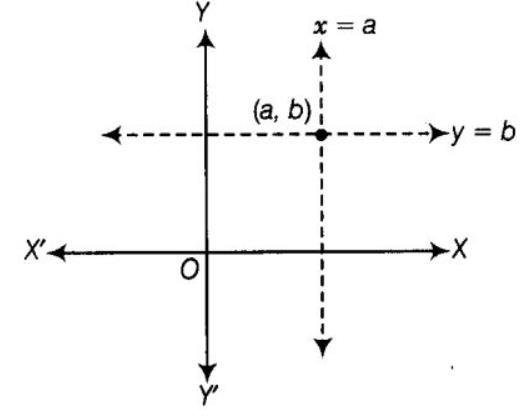Similarly, in all cases two lines intersect at (a, b).

QUESTION: 7

The sum of the digits of a two-digit number is 9. If 27 is added to it, the digit of the number gets reversed. The number is

Solution:

Lets,

First digit number = x

Second digit number = y

Number = (x+10y)

A/Q,

x + y = 9 ...................... (i)

A/Q,

(x+10y) = (10x+y) + 27

x + 10y = 10x + y +27

9x - 9y = 27

9(x - y) = 27

x - y = 27/9

x - y = 3 ......................... (ii)

Equation (i) and (ii) we get,

x = 3

Putting the value of x in eq.(i)

we get,

y = 6

Number = (10x +y)

= 10 x 3 + 6

= 30 + 6

= 36

QUESTION: 8

Match the Column: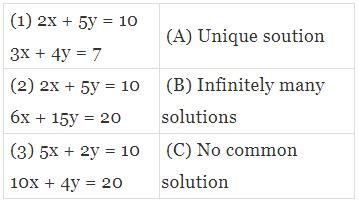Solution: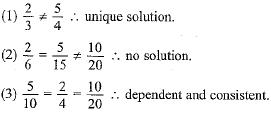QUESTION: 9

If x = a, y = b is the solution of the pair of equations x - y = 2 and x + y = 4, then the respective values of a and b are

Solution: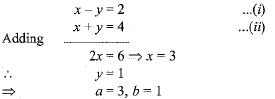QUESTION: 10

A pair of linear equations which has a unique solution x = 2, y = - 3 is

Solution:

x - 4y -14 = 0

x = 4y+14

putting this in 5x - y -13 = 0

5(4y+14) - y -13 =0

19y = -57

y = -3

putting y = -3 in x = 4y+14

x = 4(-3)+14

x = 2

so option b is correct